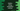# Python string endswith method explanation with example## Python string endswith() method:

The endswith method of python string is used to check if a string ends with a specific suffix or not. It returns one boolean value. If the string ends with the provided suffix, it returns True. Else, it returns False.

We can also pass start and end indices to search in the string. These are optional values. This is a useful method in Python and an easy way to check if any strings end with another string.

In this post, we will learn how to use the endswith method with different examples.

### Definition of endswith():

The endswith method is defined as like below:

``str.endswith(suffix[,start[,end]])``

This method takes three parameters.

• suffix is the suffix to search. We can also pass a tuple of strings. This value is required.
• start is an optional value. This is the index to start the search. By default, it is 0.
• end is also an optional value. This is the position to end the search i.e. up to this position, suffix will be searched in the string str.

### Return value of endswith:

The endswith method returns one boolean value. It will return True if the suffix is found in the given start and end range. Else, it will return False.

This method is case-sensitive.**

### Example of endswith() method:

Let’s take one example of endswith:

``````str_arr = ['hello world', 'hello World', 'hello worldworld',
'hello universeworld', 'world', '', 'xworld', 'xworldx']

for str in str_arr:
print(str+": ", str.endswith('world'))``````

str_arr is an array of strings. It uses a for loop to iterate through the strings and executes endswith(’world’) on each of these strings.

It will print the below output:

``````hello world:  True
hello World:  False
hello worldworld:  True
hello universeworld:  True
world:  True
:  False
xworld:  True
xworldx:  False``````

As you can see here, it returns False if it doesn’t match the end string, even if any character is in different case.

### Example of endswith() with start value:

Let’s take a look at the below example, it passes the second argument, i.e. the start value to start the search:

``````str_1 = 'hello world'

print(str_1.endswith('world', 4)) # True
print(str_1.endswith('world', 6)) # True
print(str_1.endswith('world', 7)) # False``````

The world starts at index 6 in the string str_1.

• The first one prints True because the search starts at index 4
• The second one prints True because the search starts at index 6
• The third one prints False because the search starts at index 7 but the word world starts at index 6

### Example of endswith() with end value:

We can also pass an end value to stop the search. If we pass both start and end values, it will start the search from the index start and ends at index end - 1. For example:

``````str_1 = 'hello world !!'

print(str_1.endswith('world', 4, 11)) # True
print(str_1.endswith('world', 6, 11)) # True
print(str_1.endswith('world', 6, 10)) # False``````

The word world starts at index 6 and ends at index 10.

• The first example prints True because the search starts at index 4 and ends at index 10.
• The second example prints True because the search starts at index 6 and ends at index 10.
• The third example prints False because the search starts at index 6 and ends at index 9.

### Example of endswith() with a tuple of strings:

endswith also accepts a tuple of strings and it returns True if it is True for at least one of the string in the tuple.

``````str_1 = 'hello world!!'

print(str_1.endswith(('!', '&', '*'))) # True
print(str_1.endswith(('world!!', '&', '*'))) # True
print(str_1.endswith(('x', '&', '*'))) # False``````

It returns False only if all of the strings in the tuple doesn’t ends at str_1.

### Example of endswith() to check if a string ends with one of the strings from a list:

We can’t pass a list to endswith(). But, we can convert a list to tuple and pass it to endswith as its parameter.

``````str_1 = 'hello world!!'

print(str_1.endswith(tuple(['!', '&', '*'])))  # True
print(str_1.endswith(tuple(['world!!', '&', '*'])))  # True
print(str_1.endswith(tuple(['x', '&', '*'])))  # False``````

It works similar to the above example.